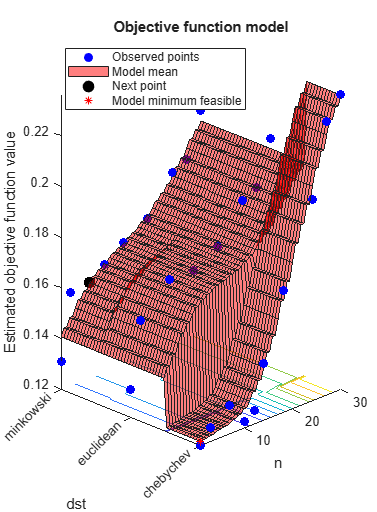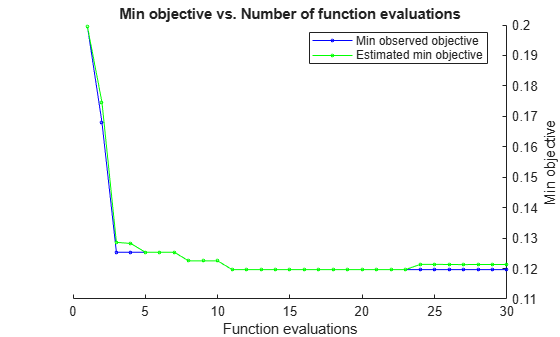# predictObjective

Predict objective function at a set of points

## Syntax

``objective = predictObjective(results,XTable)``
``````[objective,sigma] = predictObjective(results,XTable)``````

## Description

example

````objective = predictObjective(results,XTable)` returns the estimated objective function value at the points in `XTable`.```

example

``````[objective,sigma] = predictObjective(results,XTable)``` also returns estimated standard deviations.```

## Examples

collapse all

This example shows how to estimate the cross-validation loss of an optimized classifier.

Optimize a KNN classifier for the `ionosphere` data, meaning find parameters that minimize the cross-validation loss. Minimize over nearest-neighborhood sizes from 1 to 30, and over the distance functions `'chebychev'`, `'euclidean'`, and `'minkowski'`.

For reproducibility, set the random seed, and set the `AcquisitionFunctionName` option to `'expected-improvement-plus'`.

```load ionosphere rng default num = optimizableVariable('n',[1,30],'Type','integer'); dst = optimizableVariable('dst',{'chebychev','euclidean','minkowski'},'Type','categorical'); c = cvpartition(351,'Kfold',5); fun = @(x)kfoldLoss(fitcknn(X,Y,'CVPartition',c,'NumNeighbors',x.n,... 'Distance',char(x.dst),'NSMethod','exhaustive')); results = bayesopt(fun,[num,dst],'Verbose',0,... 'AcquisitionFunctionName','expected-improvement-plus');```Create a table of points to estimate.

```b = categorical({'chebychev','euclidean','minkowski'}); n = [1;1;1;4;2;2]; dst = [b(1);b(2);b(3);b(1);b(1);b(3)]; XTable = table(n,dst);```

Estimate the objective and standard deviation of the objective at these points.

```[objective,sigma] = predictObjective(results,XTable); [XTable,table(objective,sigma)]```
```ans=6×4 table n dst objective sigma _ _________ _________ _________ 1 chebychev 0.12132 0.0068029 1 euclidean 0.14052 0.0079128 1 minkowski 0.14057 0.0079117 4 chebychev 0.1227 0.0068805 2 chebychev 0.12176 0.0066739 2 minkowski 0.1437 0.0075448 ```

## Input Arguments

collapse all

Bayesian optimization results, specified as a `BayesianOptimization` object.

Prediction points, specified as a table with D columns, where D is the number of variables in the problem. The function performs its predictions on these points.

Data Types: `table`

## Output Arguments

collapse all

Objective estimates, returned as an `N`-by-`1` vector, where `N` is the number of rows of `XTable`. The estimates are the mean values of the posterior distribution of the Gaussian process model of the objective function.

Standard deviations of objective function, returned as an `N`-by-`1` vector, where `N` is the number of rows of `XTable`. The standard deviations are those of the posterior distribution of the Gaussian process model of the objective function.